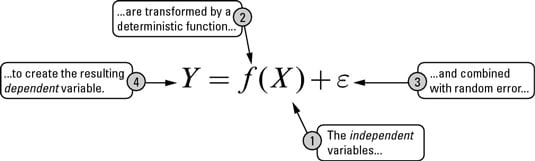##### Six Sigma For DummiesAll of Six Sigma can be summarized with what’s called the breakthrough equation — one general-purpose equation that shouldn’t intimidate even the least mathematically inclined: Y = f(X) + ε, where

• Y is the outcome(s) or result(s) you desire or need.

• X represents the inputs, factors, or pieces necessary to create the outcome(s). You can have several Xs.

• f is the function, the way or process by which the inputs are transformed into the outcome.

• ε is the presence of error, or uncertainty surrounding how accurately the Xs are transformed to create the outcome.In other words, a set of input variables is transformed by a function (or process) and combined with error to form the output. The Y results from, or is a function of, the Xs. To determine a desired outcome, you apply a transformation process or function, f, on the inputs.

You make a loaf of bread by taking flour, yeast, salt, and the other ingredients and transforming them through mixing and baking into a desired outcome. The ingredients and oven settings are the Xs, the mixing and the baking are the transformation process function f, and the resulting yummy loaf of bread is the Y.

But extra error (ε) enters in from the myriad of factors you don’t account for, like the local humidity the day you bake the bread or the freshness of the flour.

In the real world, no matter how hard you try, nuisance factors always add extra effect to the outcome; you always experience some degree of uncertainty as to how well your controlled actions produce their desired or expected result.

The Y = f(X) + ε breakthrough equation is the underlying form of all the statistical tools in Six Sigma, from correlation to curve fitting to design of experiments to hypothesis tests, and so on.

The interpretation and objective are always the same: What portion of the product or process situation’s output (the Y) can you attribute to the critical few input factors (the Xs), and how much remains in the uncertainty or error (ε)? The objective is to discover an appropriate level of knowledge regarding the Xs and their deterministic effect on Y so that only a tolerable level of ε risk remains.